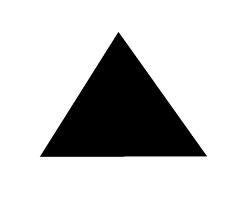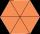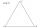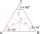# Equilateral triangle v2

Equilateral triangle has a perimeter 36 dm. What is its area?

S =  62.3538 dm2

### Step-by-step explanation:

Try calculation via our triangle calculator.Did you find an error or inaccuracy? Feel free to write us. Thank you!Tips to related online calculators
Pythagorean theorem is the base for the right triangle calculator.

#### You need to know the following knowledge to solve this word math problem:

We encourage you to watch this tutorial video on this math problem:

## Related math problems and questions:

• Triangle SASCalculate the triangle area and perimeter, if the two sides are 51 cm and 110 cm long and angle them clamped is 130 °.
• Equilateral triangleCalculate the area of an equilateral triangle with circumference 72cm.
• Circle sectionEquilateral triangle with side 33 is inscribed circle section whose center is in one of the vertices of the triangle and the arc touches the opposite side. Calculate: a) the length of the arc b) the ratio betewwn the circumference to the circle sector and
• The perimeterThe perimeter of equilateral △PQR is 12. The perimeter of regular hexagon STUVWX is also 12. What is the ratio of the area of △PQR to the area of STUVWX?
• Angle of diagonalsCalculate the perimeter and the area of a rectangle if its diagonal is 14 cm and the diagonals form an angle of 130°.
• Isosceles right triangleCalculate the area of an isosceles right triangle whose perimeter is 252 cm.
• SAS triangleThe triangle has two sides long 7 and 19 and included angle 36°. Calculate area of this triangle.
• Right triangleCalculate the missing side b and interior angles, perimeter, and area of ​​a right triangle if a=10 cm and hypotenuse c = 16 cm.
• Similarity coefficientThe ratio of similarity of two equilateral triangles is 3.5 (ie 7:2). The length of the side of smaller triangle is 2.4 cm. Calculate the perimeter and area of ​​the larger triangle.
• Equilateral triangleFind the area of an equilateral triangle with a side of 15 cm.
• TriangleCalculate the triangle sides if its area S = 630 and the second cathetus is shorter by 17.
• Similarity n-gon9-gones ABCDEFGHI and A'B'C'D'E'F'G'H'I' are similar. The area of 9-gon ABCDEFGHI is S1=190 dm2 and the diagonal length GD is 32 dm. Calculate area of the 9-gon A'B'C'D'E'F'G'H'I' if G'D' = 13 dm.
• Eq triangle minus arcsIn an equilateral triangle with a 2cm side, the arcs of three circles are drawn from the centers at the vertices and radii 1cm. Calculate the content of the shaded part - a formation that makes up the difference between the triangle area and circular cuts
• Equilateral triangleThe equilateral triangle has a 23 cm long side. Calculate its content area.
• Ratio in trapeziumThe height v and the base a, c in the trapezoid ABCD are in the ratio 1: 6: 3, its content S = 324 square cm. Peak angle B = 35 degrees. Determine the perimeter of the trapezoid
• Circle arcCircle segment has a circumference of 135.26 dm and 2096.58 dm2 area. Calculate the radius of the circle and size of the central angle.
• Equilateral triangle vs circleFind the area of an equilateral triangle inscribed in a circle of radius r = 9 cm. What percentage of the circle area does it occupy?